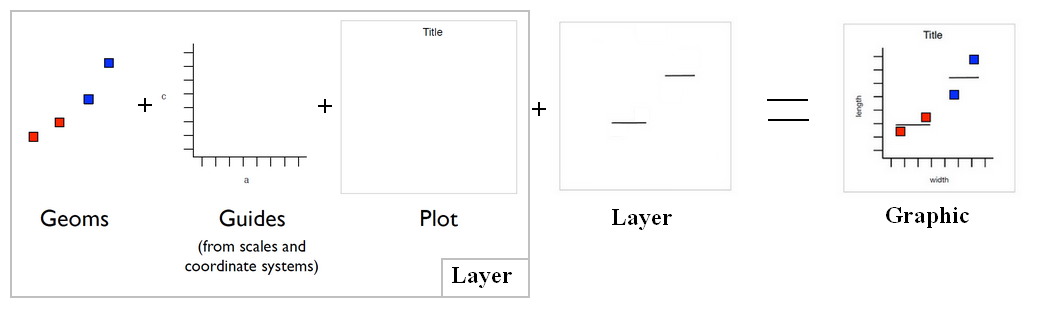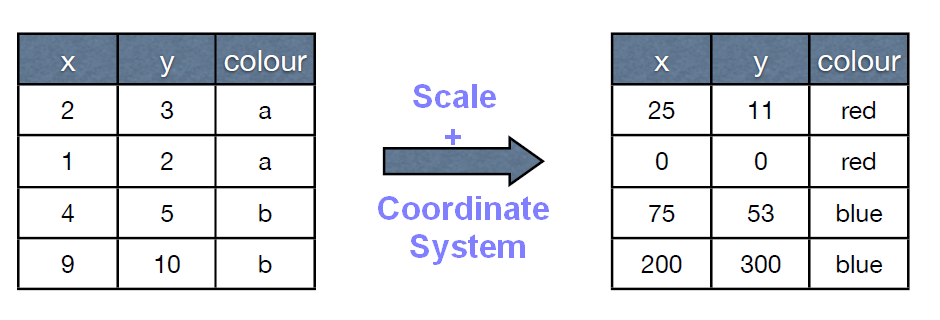# Ggplot - (aes|aesthetic) (plot parameter definition)

Aesthetic are plots parameters that are given by the data.

For parameters that are not related to the data, see Ggplot - Theme

Such as:

• coordinate x and y.
• Color:
• color: the bar outline,
• fill: interior colouring
• alpha
• group - How the data are grouped (Default to the discrete value of the plot)
• weight - The variable to be summed.
• shape - It will use another shape for each point

The aes function maps aesthetic to data frame variable.

## Syntax

````mapping = aes(x=carat, y=price)`
```

The aes function (map|set):

• the variable carat to x
• and the variable price to y.

Mappings usually stay the same on a plot, so they are stored as defaults

### Example

``````# The carat variable will be x and the prices will be y
p <- ggplot(diamonds, aes(x=carat,y=price))

# This code will map the colour to the green variable
p + geom_point(aes(colour = "green"))

# This code will show the colour green for all geoms
p + geom_point(colour = "green")```
```
``````ggplot(diamonds, aes(x=carat, y=price))
+ geom_point(aes(colour = carat))```
```
• fill. To slice an histogram, area with colour.
``````ggplot(diamonds, aes(x=price))
+geom_histogram(aes(fill = clarity))```
```

Recommended PagesGGplot - Layer (data + mapping + geom + stat + position)

Layer = data: The data frame (in a variable or a function, if null inherited) + mapping: Mapping data to geom properties (ie aesthetics) + geom : The geometric object to use display the data +...GGplot - Scale (Data Transformation)

Scales control the mapping between data and aesthetics, and control the display of the matching guide (axis or legend) Ex: Linear scaling of x and y axes (scales) See: scale_continuous, scale_discrete,...GGplot - Stat - (Statistical transformation|Statistic)

The Statistical transformation (stat). Multiple layers, statistical transformation. It's often useful to transform your data before plotting, and that's what statistical transformations do. Every...Ggplot - Color

Colour is an aesthetic (plot parameter) that changes the bar outline color. See also: fill to change the interior colouring. alpha for the transparency A name, e.g., “red”. R has 657 built-in...Ggplot - Data

Data in the context of Ggplot refers to the (source) data of the data frame. The aesthetic method maps: the data from the data frame (data) to the data of the plot (an aesthetic). See Data...Ggplot - Fill aesthetic

Fill in Ggplot Fill is an aesthetic and changes the interior coloring. See: color for the bar outline alpha for the transparencyGgplot - Order

Order in Ggplot A factor is plotted ordered by its level, you can reorder it with the reorder function. By default, the order is alphabetical, The plot is not ordered by x but by the alphabet...Ggplot - Plot Parameters

For parameters, related to: the data: see the whole plot: seeGgplot - Support

“” This error occurs when ggplot doesn't no the x and y aesthetic. Resolution: when defining a plot, define it with the aesthetic x and y by defaultGgplot - Weight aesthetic

The weight aesthetic represents generally a variable that must be summed.